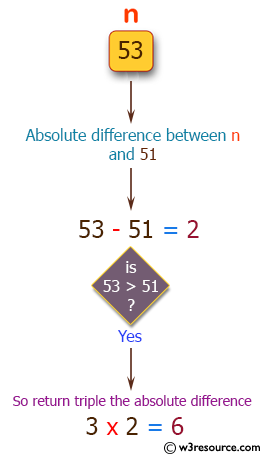﻿ Swift Basic Programming Exercise: Compute and return the absolute difference of n and 51 - w3resource# Swift Basic Programming Exercise: Compute and return the absolute difference of n and 51

## Swift Basic Programming: Exercise-2 with Solution

Write a Swift program to compute and return the absolute difference of n and 51, if n is over 51 return double the absolute difference.

Pictorial Presentation:Sample Solution:

Swift Code:

``````func diff_51(x: Int) -> Int {
if x > 51
{
return (x - 51) * 2
}
else
{
return 51 - x
}
}

print(diff_51(x: 45))
print(diff_51(x: 61))
print(diff_51(x: 21))
```
```

Sample Output:

```6
20
30
```

Swift Programming Code Editor:

Improve this sample solution and post your code through Disqus

What is the difficulty level of this exercise?

﻿